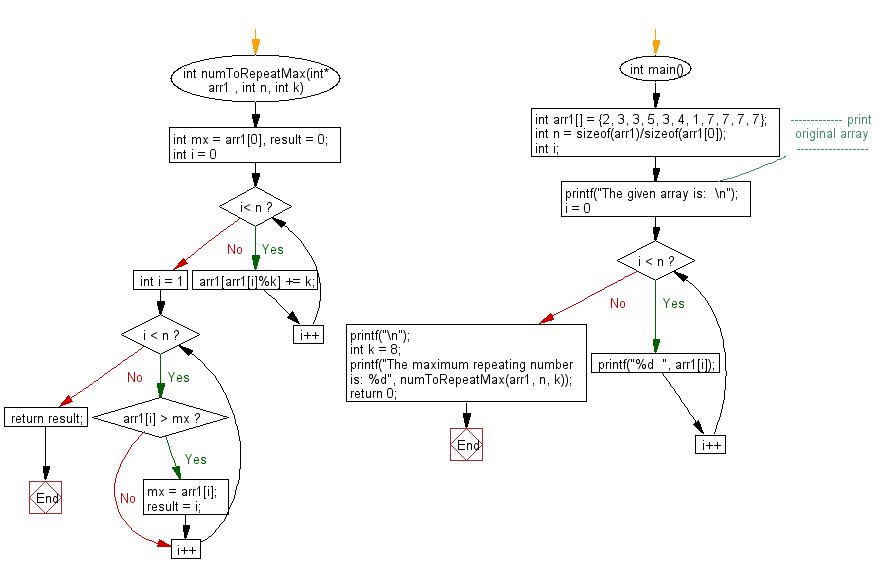﻿ C exercises: Find the maximum repeating number in a given array - w3resource# C Exercises: Find the maximum repeating number in a given array

## C Array: Exercise-81 with Solution

Write a program in C to find the maximum repeating number in a given array. The array range is [0..n-1] and the elements are in the range [0..k-1] and k<=n.

Sample Solution:

C Code:

``````#include <stdio.h>
int numToRepeatMax(int* arr1 , int n, int k)
{
int mx = arr1, result = 0;
for (int i = 0; i< n; i++)
arr1[arr1[i]%k] += k;

for (int i = 1; i < n; i++)
{
if (arr1[i] > mx)
{
mx = arr1[i];
result = i;
}
}
return result;
}

int main()
{
int arr1[] = {2, 3, 3, 5, 3, 4, 1, 7, 7, 7, 7};
int n = sizeof(arr1)/sizeof(arr1);
int i;
//------------- print original array ------------------
printf("The given array is:  \n");
for(i = 0; i < n; i++)
{
printf("%d  ", arr1[i]);
}
printf("\n");
//------------------------------------------------------
int k = 8;
printf("The maximum repeating number is: %d", numToRepeatMax(arr1, n, k));
return 0;
}
```
```

Sample Output:

```The given array is:
2  3  3  5  3  4  1  7  7  7  7
The maximum repeating number is: 7
```

Flowchart:C Programming Code Editor:

Improve this sample solution and post your code through Disqus.

﻿